# Calculate the time in which the electron would acquire a velocity double of its initial velocity

An electron moving with a velocity of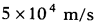enters into a uniform electric field and acquires a uniform acceleration of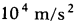in the direction of its initial motion. Calculate the time in which the electron would acquire a velocity double of its initial velocity.

Given,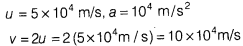From first equation of motion, v = u + at
i.e,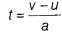=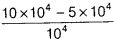=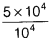Thus, the time in which electron acquire a velocity double of its initial velocity is 5 seconds.